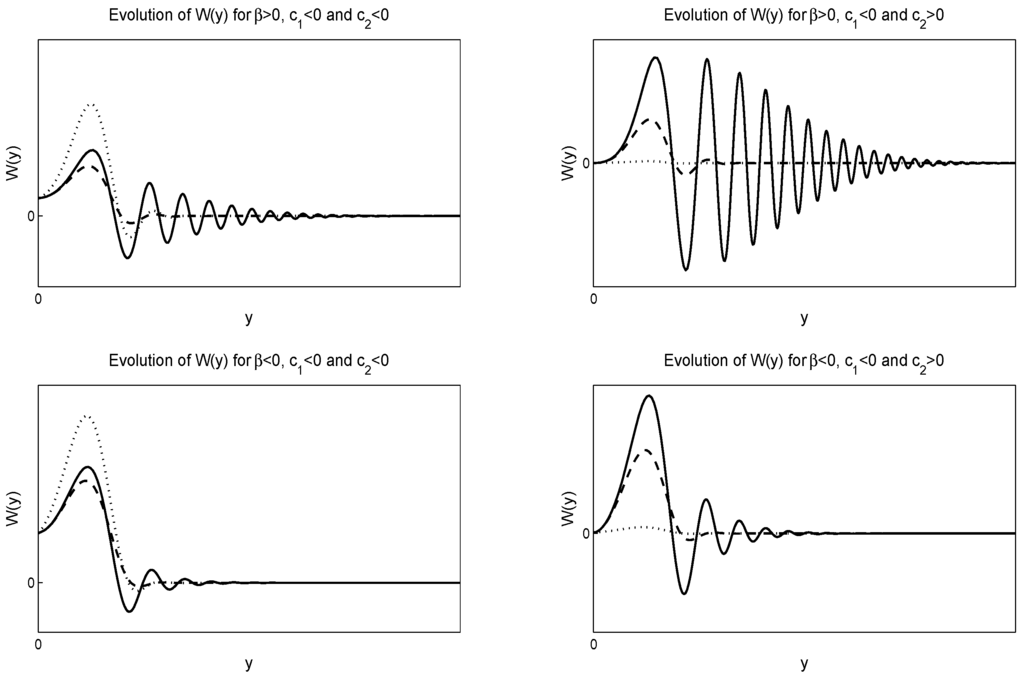Next Article in Journal
Fractional Schrödinger Equation in the Presence of the Linear Potential
Next Article in Special Issue
Lie Symmetries of (1+2) Nonautonomous Evolution Equations in Financial Mathematics
Previous Article in Journal
An Adaptive WENO Collocation Method for Differential Equations with Random Coefficients
Previous Article in Special Issue
Barrier Option Under Lévy Model : A PIDE and Mellin Transform Approach
Article

# Lie Symmetry Analysis of the Black-Scholes-Merton Model for European Options with Stochastic Volatility

1
Instituto de Ciencias Físicas y Matemáticas, Universidad Austral de Chile, Valdivia 5090000, Chile
2
Department of Mathematics, Pondicherry University, Kalapet 605014, India
3
Institute of Systems Science, Department of Mathematics, Durban University of Technology, Durban 4000, South Africa
4
School of Mathematics, Statistics and Computer Science, University of KwaZulu-Natal, Durban 4000, South Africa
5
Department of Mathematics and Statistics, University of Cyprus, Lefkosia 1678, Cyprus
*
Author to whom correspondence should be addressed.
These authors contributed equally to this work.
Mathematics 2016, 4(2), 28; https://doi.org/10.3390/math4020028
Received: 30 January 2016 / Revised: 14 April 2016 / Accepted: 15 April 2016 / Published: 3 May 2016
We perform a classification of the Lie point symmetries for the Black-Scholes-Merton Model for European options with stochastic volatility, σ, in which the last is defined by a stochastic differential equation with an Orstein-Uhlenbeck term. In this model, the value of the option is given by a linear (1 + 2) evolution partial differential equation in which the price of the option depends upon two independent variables, the value of the underlying asset, S, and a new variable, y. We find that for arbitrary functional form of the volatility, $σ ( y )$ , the (1 + 2) evolution equation always admits two Lie point symmetries in addition to the automatic linear symmetry and the infinite number of solution symmetries. However, when $σ ( y ) = σ 0$ and as the price of the option depends upon the second Brownian motion in which the volatility is defined, the (1 + 2) evolution is not reduced to the Black-Scholes-Merton Equation, the model admits five Lie point symmetries in addition to the linear symmetry and the infinite number of solution symmetries. We apply the zeroth-order invariants of the Lie symmetries and we reduce the (1 + 2) evolution equation to a linear second-order ordinary differential equation. Finally, we study two models of special interest, the Heston model and the Stein-Stein model. View Full-Text
Show FiguresFigure 1

MDPI and ACS Style

Paliathanasis, A.; Krishnakumar, K.; Tamizhmani, K.M.; Leach, P.G.L. Lie Symmetry Analysis of the Black-Scholes-Merton Model for European Options with Stochastic Volatility. Mathematics 2016, 4, 28. https://doi.org/10.3390/math4020028

AMA Style

Paliathanasis A, Krishnakumar K, Tamizhmani KM, Leach PGL. Lie Symmetry Analysis of the Black-Scholes-Merton Model for European Options with Stochastic Volatility. Mathematics. 2016; 4(2):28. https://doi.org/10.3390/math4020028

Chicago/Turabian Style

Paliathanasis, Andronikos, K. Krishnakumar, K.M. Tamizhmani, and Peter G.L. Leach 2016. "Lie Symmetry Analysis of the Black-Scholes-Merton Model for European Options with Stochastic Volatility" Mathematics 4, no. 2: 28. https://doi.org/10.3390/math4020028

Find Other Styles
Note that from the first issue of 2016, MDPI journals use article numbers instead of page numbers. See further details here.

1